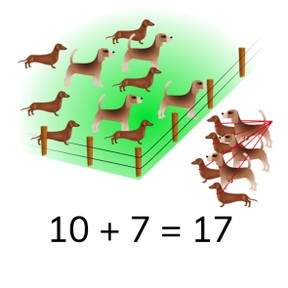# Recognizing addition problems to 20

No account needed.8,000 schools use Gynzy92,000 teachers use Gynzy1,600,000 students use Gynzy

## General

Students learn to match an image to an addition problem with numbers up to 20.

## Introduction

Set a few blocks on the table at the front of the class (maximum of 20). Ask students to come to the front and ask how many blocks are on the table. Repeat this with pencils. Show the cups on the interactive whiteboard and ask the class to count how many there are. Ask students if there are tricks or ways to count that are easier than counting one by one. Say that you can cross off the objects as you count them. Then count the rugby balls.

## Development

Using the baby chicks, explain that if you know the addition problem, you can also solve for the sum or total by counting the two addends together. Practice this with the pencils by having students first find the addition problem, and then solving it. Repeat this with the image of flowers.

Check that students understand recognizing addition problems to 20 by asking the following questions:
- How do you know which numbers make up an addition problem?
- What do you do if you want to know the total of both groups of objects?
- How do you know what the first addend in an addition problem is?

## Guided practice

Students first practice recognizing addition problems from provided answers in multiple-choice. Then they must match the image to a given addition problem. Finally, they must give the addition problem as well as solve the problem.

## Closing

Discuss with students that it is important to be able to recognize addition problems up to 20, so you can learn how to add. Next ask a student to come to the front of the class and secretly show them an abstract addition problem. Ask them to draw an image/objects to match the shown addition problem. They can draw circles, squares, butterflies, flowers, or more. Make sure that the student makes a clear division between the group representing the first addend and the group representing the second addend. After the student has finished drawing, then ask the class if they can determine what the addition problem is, and then solve for the sum.

## Teaching tips

Students who have difficulty adding using images can be supported by the use of manipulatives like MAB-blocks. Have them set one group of manipulatives to the left, the other to the right, and then to count the objects one by one.

## Instruction materials

blocks and pencils

### The online teaching platform for interactive whiteboards and displays in schools

• Save time building lessons

• Manage the classroom more efficiently

• Increase student engagement